### Home > PC > Chapter 4 > Lesson 4.2.3 > Problem4-104

4-104.
1.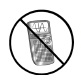Find the exact value of each of the following trig expressions without using a calculator. Homework Help ✎

1. cos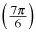2. sin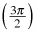3. sec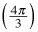4. tan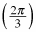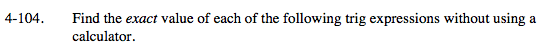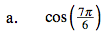Draw a unit circle with reference angle.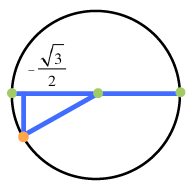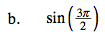Follow the hint in part (a).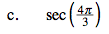$\sec(\theta)=\frac{1}{\cos(\theta)}$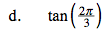Follow the hint in part (a).